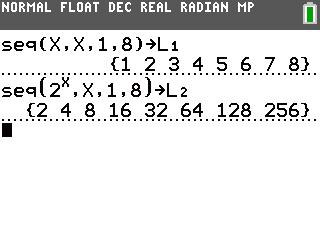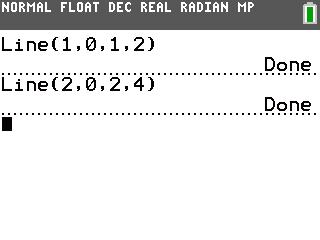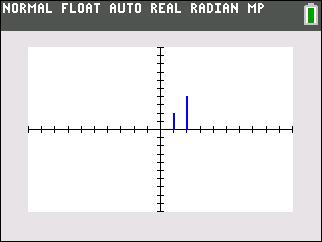# Activities

••• ##### Subject Area

• Math: Algebra I: Sequences and Series

• ##### Author9-12

30 Minutes

• ##### Device
• TI-83 Plus Family
• TI-84 Plus
• TI-84 Plus Silver Edition
•TI-84 Plus C Silver Edition
•TI-84 Plus CE
• ##### Report an Issue

Double Tree#### Activity Overview

Students visually explore geometric sequences by modeling the growth of a tree that doubles in height every year.

#### Key Steps

•In this activity, students model tree growth using the sequence 2n. They use the Line command to draw line segments, representing the height of a tree. The commands shown in the screenshot, draws lines from (1, 0) to (1, 2) and (2, 0) to (2, 4).

•Students complete a table describing the x-value of the line, height of the tree, and the power of two for the years 2001 to 2008.Students complete a table describing the x-value of the line, height of the tree, and the power of two for the years 2001 to 2008.

•Then students use the seq command to create a scatter plot the sequence 2n. The first sequence, stored in L1, contains the integers 1 to 8, representing the years. The second sequence, stored in L2, contains the values of the sequence 2n, representing the heights of the tree.